# Unit iii measures of dispersion (2)

16 de Oct de 2021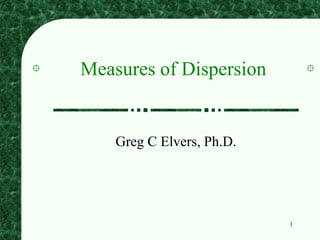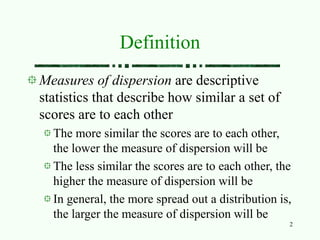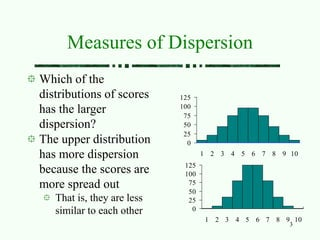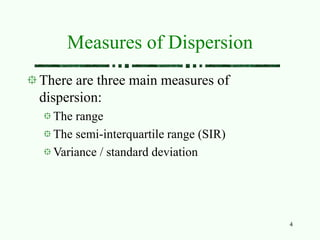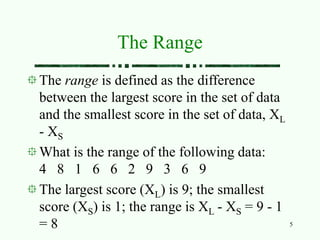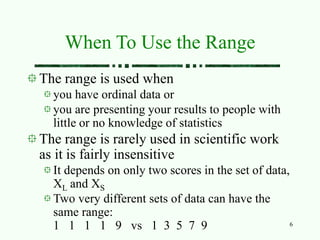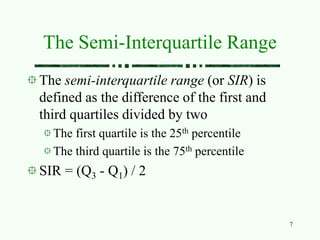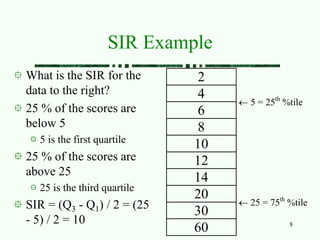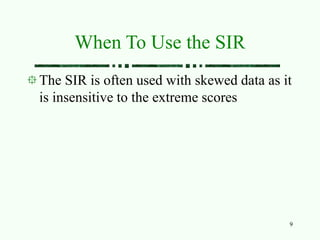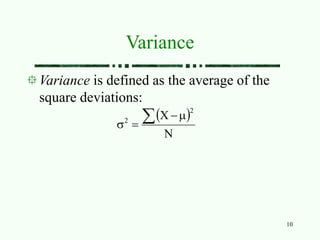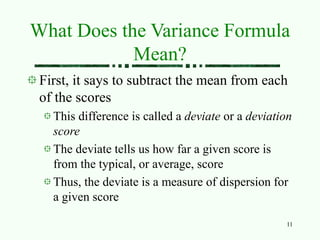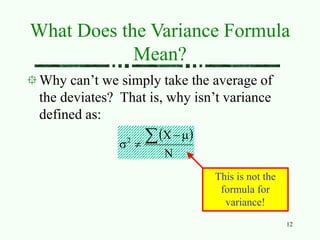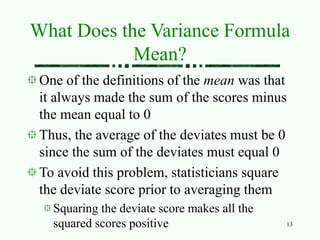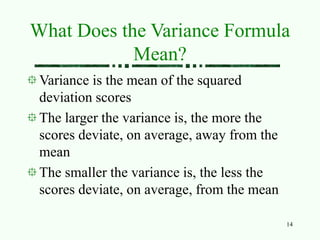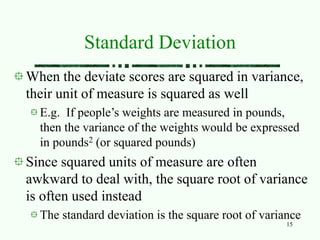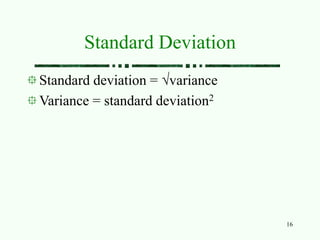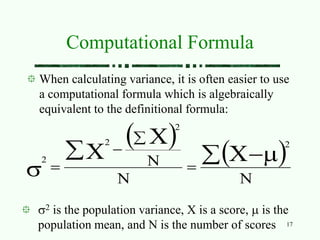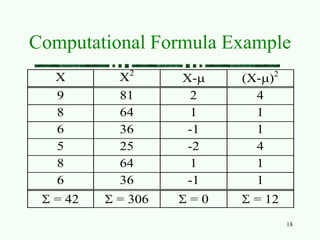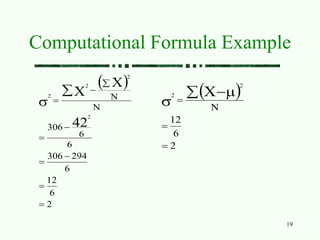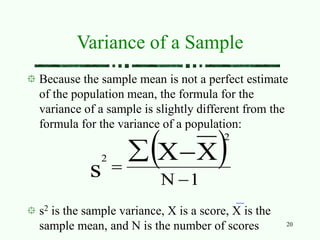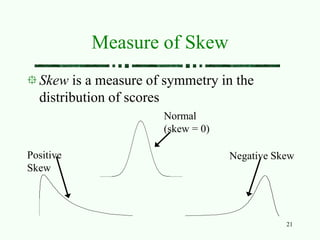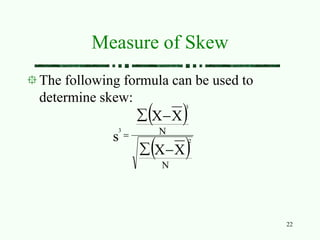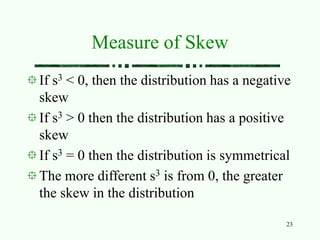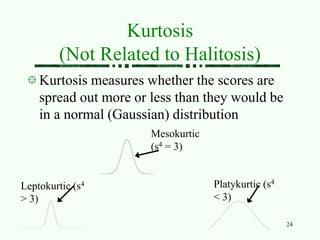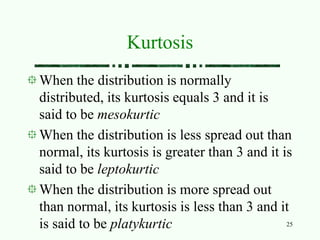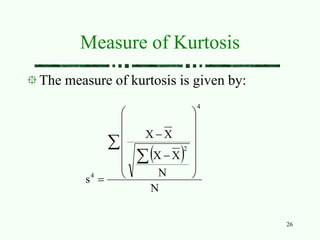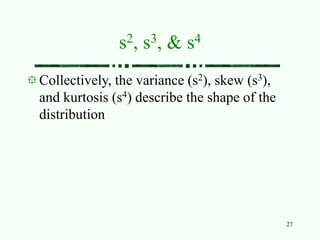1 de 27

### Unit iii measures of dispersion (2)

• 1. 1 Measures of Dispersion Greg C Elvers, Ph.D.
• 2. 2 Definition Measures of dispersion are descriptive statistics that describe how similar a set of scores are to each other The more similar the scores are to each other, the lower the measure of dispersion will be The less similar the scores are to each other, the higher the measure of dispersion will be In general, the more spread out a distribution is, the larger the measure of dispersion will be
• 3. 3 Measures of Dispersion Which of the distributions of scores has the larger dispersion? 0 25 50 75 100 125 1 2 3 4 5 6 7 8 9 10 0 25 50 75 100 125 1 2 3 4 5 6 7 8 9 10 The upper distribution has more dispersion because the scores are more spread out That is, they are less similar to each other
• 4. 4 Measures of Dispersion There are three main measures of dispersion: The range The semi-interquartile range (SIR) Variance / standard deviation
• 5. 5 The Range The range is defined as the difference between the largest score in the set of data and the smallest score in the set of data, XL - XS What is the range of the following data: 4 8 1 6 6 2 9 3 6 9 The largest score (XL) is 9; the smallest score (XS) is 1; the range is XL - XS = 9 - 1 = 8
• 6. 6 When To Use the Range The range is used when you have ordinal data or you are presenting your results to people with little or no knowledge of statistics The range is rarely used in scientific work as it is fairly insensitive It depends on only two scores in the set of data, XL and XS Two very different sets of data can have the same range: 1 1 1 1 9 vs 1 3 5 7 9
• 7. 7 The Semi-Interquartile Range The semi-interquartile range (or SIR) is defined as the difference of the first and third quartiles divided by two The first quartile is the 25th percentile The third quartile is the 75th percentile SIR = (Q3 - Q1) / 2
• 8. 8 SIR Example What is the SIR for the data to the right? 25 % of the scores are below 5 5 is the first quartile 25 % of the scores are above 25 25 is the third quartile SIR = (Q3 - Q1) / 2 = (25 - 5) / 2 = 10 2 4 6  5 = 25th %tile 8 10 12 14 20 30  25 = 75th %tile 60
• 9. 9 When To Use the SIR The SIR is often used with skewed data as it is insensitive to the extreme scores
• 10. 10 Variance Variance is defined as the average of the square deviations:   N X 2 2     
• 11. 11 What Does the Variance Formula Mean? First, it says to subtract the mean from each of the scores This difference is called a deviate or a deviation score The deviate tells us how far a given score is from the typical, or average, score Thus, the deviate is a measure of dispersion for a given score
• 12. 12 What Does the Variance Formula Mean? Why can’t we simply take the average of the deviates? That is, why isn’t variance defined as:   N X 2      This is not the formula for variance!
• 13. 13 What Does the Variance Formula Mean? One of the definitions of the mean was that it always made the sum of the scores minus the mean equal to 0 Thus, the average of the deviates must be 0 since the sum of the deviates must equal 0 To avoid this problem, statisticians square the deviate score prior to averaging them Squaring the deviate score makes all the squared scores positive
• 14. 14 What Does the Variance Formula Mean? Variance is the mean of the squared deviation scores The larger the variance is, the more the scores deviate, on average, away from the mean The smaller the variance is, the less the scores deviate, on average, from the mean
• 15. 15 Standard Deviation When the deviate scores are squared in variance, their unit of measure is squared as well E.g. If people’s weights are measured in pounds, then the variance of the weights would be expressed in pounds2 (or squared pounds) Since squared units of measure are often awkward to deal with, the square root of variance is often used instead The standard deviation is the square root of variance
• 16. 16 Standard Deviation Standard deviation = variance Variance = standard deviation2
• 17. 17 Computational Formula When calculating variance, it is often easier to use a computational formula which is algebraically equivalent to the definitional formula:     N N N X X X 2 2 2 2          2 is the population variance, X is a score,  is the population mean, and N is the number of scores
• 18. 18 Computational Formula Example X X2 X- (X-)2 9 81 2 4 8 64 1 1 6 36 -1 1 5 25 -2 4 8 64 1 1 6 36 -1 1  = 42  = 306  = 0  = 12
• 19. 19 Computational Formula Example   2 6 12 6 294 306 6 6 306 N N 42 X X 2 2 2 2              2 6 12 N X 2 2       
• 20. 20 Variance of a Sample Because the sample mean is not a perfect estimate of the population mean, the formula for the variance of a sample is slightly different from the formula for the variance of a population:   1 N X X s 2 2     s2 is the sample variance, X is a score, X is the sample mean, and N is the number of scores
• 21. 21 Measure of Skew Skew is a measure of symmetry in the distribution of scores Positive Skew Negative Skew Normal (skew = 0)
• 22. 22 Measure of Skew The following formula can be used to determine skew:     N N X X X X s 2 3 3     
• 23. 23 Measure of Skew If s3 < 0, then the distribution has a negative skew If s3 > 0 then the distribution has a positive skew If s3 = 0 then the distribution is symmetrical The more different s3 is from 0, the greater the skew in the distribution
• 24. 24 Kurtosis (Not Related to Halitosis) Kurtosis measures whether the scores are spread out more or less than they would be in a normal (Gaussian) distribution Mesokurtic (s4 = 3) Leptokurtic (s4 > 3) Platykurtic (s4 < 3)
• 25. 25 Kurtosis When the distribution is normally distributed, its kurtosis equals 3 and it is said to be mesokurtic When the distribution is less spread out than normal, its kurtosis is greater than 3 and it is said to be leptokurtic When the distribution is more spread out than normal, its kurtosis is less than 3 and it is said to be platykurtic
• 26. 26 Measure of Kurtosis The measure of kurtosis is given by:   N N X X X X s 4 2 4                   
• 27. 27 s2, s3, & s4 Collectively, the variance (s2), skew (s3), and kurtosis (s4) describe the shape of the distribution